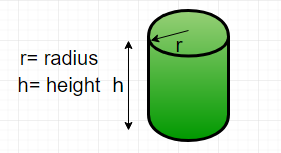# Java Program for Find the perimeter of a cylinder

• Difficulty Level : Medium
• Last Updated : 22 Jun, 2022

Given diameter and height, find the perimeter of a cylinder. Perimeter is the length of the outline of a two – dimensional shape. A cylinder is a three – dimensional shape. So, technically we cannot find the perimeter of a cylinder but we can find the perimeter of the cross-section of the cylinder. This can be done by creating the projection on its base, thus, creating the projection on its side, then the shape would be reduced to a rectangle.Formula : Perimeter of cylinder ( P ) =here d is the diameter of the cylinder h is the height of the cylinder Examples :

```Input : diameter = 5, height = 10
Output : Perimeter = 30

Input : diameter = 50, height = 150
Output : Perimeter = 400```

## Java

 `// Java program to find``// perimeter of cylinder``import` `java.io.*;` `class` `GFG {` `    ``// Function to calculate perimeter``    ``static` `int` `perimeter(``int` `diameter, ``int` `height)``    ``{``        ``return` `2` `* (diameter + height);``    ``}``    ` `    ``/* Driver program to test above function */``    ``public` `static` `void` `main(String[] args)``    ``{``        ``int` `diameter = ``5``;``        ``int` `height = ``10``;``        ``System.out.println(``"Perimeter = "` `+``                        ``perimeter(diameter, height)``                                    ``+ ``" units\n"``);``    ``}``}` `// This code is contributed by Gitanjali.`

Output :

`Perimeter = 30 units`

Time complexity: O(1)

Auxiliary Space: O(1)

Please refer complete article on Find the perimeter of a cylinder for more details!

My Personal Notes arrow_drop_up